# Unitriangular matrix group

## Definition

### Definition as matrix group

Suppose$R$ is a commutative unital ring and$n$ is a natural number. The unitriangular matrix group, denoted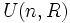$U(n,R)$,$UT(n,R)$, or$UL(n,R)$, is the group, under multiplication, with$1$s on the diagonal,$0$s below the diagonal, and arbitrary entries above the diagonal.

Note that the symbol$U$ is also used for the unitary group, hence we use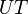$UT$ or$UL$to avoid confusion.

Explicitly: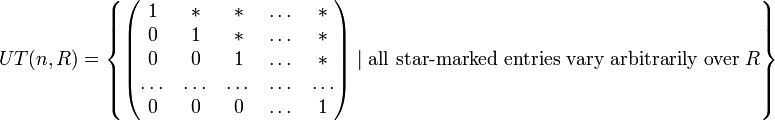$UT(n,R) = \left \{ \begin{pmatrix} 1 & * & * & \dots & * \\ 0 & 1 & * & \dots & * \\ 0 & 0 & 1 & \dots & * \\ \dots & \dots & \dots & \dots & \dots \\ 0 & 0 & 0 & \dots & 1 \\\end{pmatrix} \mid \mbox{all star-marked entries vary arbitrarily over } R \right \}$

### Definition by presentation

The presentation given here is similar to the presentation used for the Steinberg group over a unital ring. Specifically, we use only those generators and relations that correspond to upper triangular matrices and discard the rest. We denote by$e_{ij}(\lambda)$ the matrix with 1s on the diagonal,$\lambda$ in the$(i,j)^{th}$ entry, and zeros elsewhere. Then:

• The following is a generating set: For every element$\lambda \in R$ and for$1 \le i , include the element$e_{ij}(\lambda)$.
• We can get a presentation of the group using this generating set, by including the following relations:
•$e_{ij}(\lambda)e_{ij}(\mu) = e_{ij}(\lambda + \mu)$ (note that this in particular implies that$e_{ij}(0)$ is the identity element for all$i,j$).
•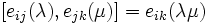$[e_{ij}(\lambda),e_{jk}(\mu)] = e_{ik}(\lambda \mu)$ (the Steinberg group version also imposes the condition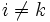$i \ne k$, but this constraint follows automatically in the upper triangular case because$i < j$ and$j < k$).
•$[e_{ij}(\lambda),e_{kl}(\mu)] = 1$ (i.e., is the identity element) for$i \ne l, j \ne k$.

Note that this presentation can be trimmed quite a bit. In fact, if$A$ is a generating set for the additive group of$R$, the set: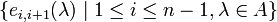$\{ e_{i,i+1}(\lambda) \mid 1 \le i \le n - 1, \lambda \in A \}$

is a generating set for$UT(n,R)$, and we can work out a presentation in terms of this generating set using the relations above.

### Case of a field

When$F$ is a field, the unitriangular matrix group$UT(n,F)$ can also be described as a maximal unipotent subgroup of the general linear group$GL(n,F)$. It is also a maximal unipotent subgroup of the special linear group$SL(n,F)$.

### Case of a finite field

When$F$ is a finite field with$q$ elements and characteristic$p$ (so$q$ is a power of$p$), then$UT(n,F)$ is also denoted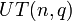$UT(n,q)$, and is a$p$-Sylow subgroup of$GL(n,F) = GL(n.q)$.

## Particular cases

Value of$n$$n(n-1)/2$ (the "dimension" of$UT(n,R)$, the size is$|R|^{n(n-1)/2}$) Nature of unitriangular matrix group$UT(n,R)$ over a ring$R$
1 0 trivial group
2 1 isomorphic to the additive group of$R$
3 3 see unitriangular matrix group of degree three
4 6 see unitriangular matrix group of degree four

## Arithmetic functions

We give here the arithmetic functions for$UT(n,R)$.

Function Value Similar groups Explanation
order$|R|^{n(n-1)/2}$
nilpotency class$n - 1$
derived length$\lceil \log_2n \rceil$ (the smallest integer greater than or equal to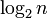$\log_2 n$
Frattini length PLACEHOLDER FOR INFORMATION TO BE FILLED IN: [SHOW MORE] (depends both on the nature of$R$ and on$n$)